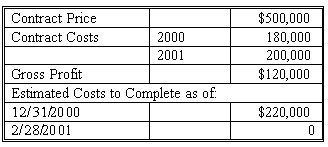### CFA Practice Question

There are 534 practice questions for this study session.

### CFA Practice Question

The following information applies to Smith Construction's Job 713, which was completed in 2001.If Smith Construction had used the completed contract method to recognize revenue, what amount of gross profit would have been recognized each year? (2000; 2001)

A. \$0; \$100,000
B. \$45,000; \$55,000
C. \$100,000; \$0

The entire \$100,000 in gross profit [500,000 - 180,000 - 220,000] would be recognized in 2001 (the year of completion).

User Comment
kalps Surely, the goross profit of 129k should be recognised in 2001 not the incorrect estimate amount - am i going mad ??
shasha i'm afraid year 2001 cost of 200k had been estimated BEFORE the revised cost of 220k. the answer is correct.
jwp2 Estimated costs play no role in the completed contract method. The answer is \$120K.
quincy the answer is correct, the newly estimated cost for 2001 is 220k, so total cost would be 400k.
morpheus918 Sorry Sasha and Quincy. But \$120k is the profit for the completed project. Therefore, that is what is recognized, not the incorrect estimate from halfway through the project.
db28luke Total costs at completion were only \$380M. I agree that gross profit should be \$120M not \$100M. The other \$20M of estimated costs were never incurred.
mtcfa I think the question writers should clarify that the top half of the question is the original estimate, and the bottom half is the revised estimate of remaining costs after incurring year 1 costs of \$180k. Then the question makes sense. Otherwise the answer should be 120k.
okoth Surely the actual costs incurred should have been used.
mordja If the question had been, "what was the expected Gross Profit to be recognized each year?" it would make sense. However given no profit would be recognized until actual cash was received they would know full well the actual GP was 120,000, not the expected \$100,000. Answer is 120,000.
Murrayman The estimated cost for year two remains \$220,000. Therefore the gross profit upon completion remains \$100,000. Please note that the original year 2(2001) cost of \$200,000 is alos estimated.
jainrajeshv No Murrayman, i am not agreed with Murrayman. 200,000 is a actual cost and not extimate. and the profit should be 120,000 for the year 2001.
bantoo I could not understand the table. Can anyone make it more clear?
hardig under completed contract method, no gross profit claimed until completed - therefore, althought here is some question about the actual vs estimate cost, there was no reason to do the calculation as only one answer had \$0 in the first year, so it could be the only correct choice.
judylyh so is the answer 100k or 120k?
johntan1979 Agree with hardig. I'm going with \$100K. In the event of confusing options, choose the best available answer.
gill15 The answer is correct. The costs have just been revised at the new date and you MUST use the new cost of 220 000 instead of 200 000 when finding your Contract Costs ----> GP = 5000000 - 180000 - 220000 = 100 g's
johntan1979 To clarify my comment, I meant I'm going with the answer A as the best answer, but I agree with most people here that the GP to be recognized in 2001 under the completed contract method should be \$120k, NOT \$100k. Why use the estimate if you only recognize profit when it is completed since you will already have the actual costs at completion?
davcer Without calculations, the only option that recognizes all revenues when the project has been completed is A
Yrazzaq88 I answered this question in 5 seconds literally, just because under the completion method, the gross profit is generally recognized at the end of the contract period.
Anacleto90 It is not clear it the Contract Costs for 2001 of 200,000 are Actual or Estimated.
praneetb A is the closest answer but 120k is the correct answer. Whats the point of using the completion of contract method if you are going to use estimates. (Because one of the conditions of use it are that estimates are unreliable therefore you wait for the contract to complete so you know what the true costs are).